﻿ Kalman Filter in one dimension

# Kalman Filter in one dimension

In this chapter, we derive the Kalman Filter in one dimension. The main goal of this chapter is to explain the Kalman Filter concept simply and intuitively without using math tools that may seem complex and confusing.

We are going to advance toward the Kalman Filter equations step by step.

In this chapter, we derive the Kalman Filter equations without process noise. In the following chapter, we add process noise.

## One-dimensional Kalman Filter without process noise

As I mentioned earlier, the Kalman Filter is based on five equations. We are already familiar with two of them:

• The state update equation
• The dynamic model equation

In this chapter, we derive another three Kalman Filter Equations and revise the state update equation.

Like the $$\alpha -\beta -(\gamma)$$ filter, the Kalman filter utilizes the "Measure, Update, Predict" algorithm.

Contrary to the $$\alpha -\beta -(\gamma)$$ filter, the Kalman Filter treats measurements, current state estimation, and next state estimation (predictions) as normally distributed random variables. The random variable is described by mean and variance.

The following chart provides a low-level schematic description of the Kalman Filter algorithm: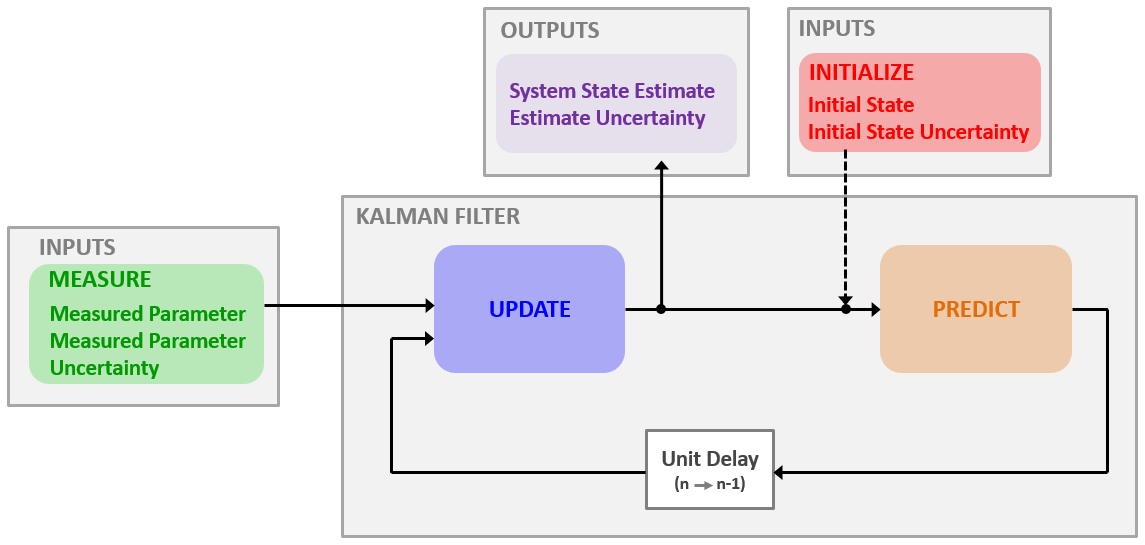Let's recall our first example (gold bar weight measurement); We made multiple measurements and computed the estimate by averaging.

We obtained the following result:The above plot shows the true, measured, and estimated values vs. the number of measurements.

### Estimate as a random variable

The difference between the estimates (the red line) and the true values (the green line) is the estimate error. As you can see, the estimate error becomes lower as we make additional measurements, and it converges towards zero, while the estimated value converges towards the true value. We don't know the estimate error, but we can estimate the state uncertainty.

We denote the state estimate variance by $$p$$.

### Measurement as a random variable

The measurement errors are the differences between the measurements (blue samples) and the true values (green line). Since the measurement errors are random, we can describe them by variance ( $$\sigma ^{2}$$ ). The standard deviation $$\left( \sigma \right)$$ of the measurement errors is the measurement uncertainty.

Note: In some literature, the measurement uncertainty is also called the measurement error.

We denote the measurement variance by $$r$$.

The variance of the measurement errors could be provided by the measurement equipment vendor, calculated, or derived empirically by a calibration procedure.

For example, when using scales, we can calibrate the scales by making multiple measurements of an item with a known weight and empirically derive the standard deviation. The scales vendor can also supply the measurement uncertainty parameter.

For advanced sensors like radar, the measurement uncertainty depends on several parameters such as SNR (Signal to Noise Ratio), beam width, bandwidth, time on target, clock stability, and more. Every radar measurement has a different SNR, beam width, and time on target. Therefore, the radar calculates the uncertainty of each measurement and reports it to the tracker.

Let's look at the weight measurements PDF (Probability Density Function).

The following plot shows ten measurements of the gold bar weight.

• The blue circles describe the measurements.
• The true values are described by the red dashed line.
• The green line describes the probability density function of the measurement.
• The bold green area is the standard deviation ( $$\sigma$$ ) of the measurement, i.e., there is a probability of 68.26% that the measurement value lies within this area.

As you can see, 7 out of 10 measurements are within $$1 \sigma$$ boundaries.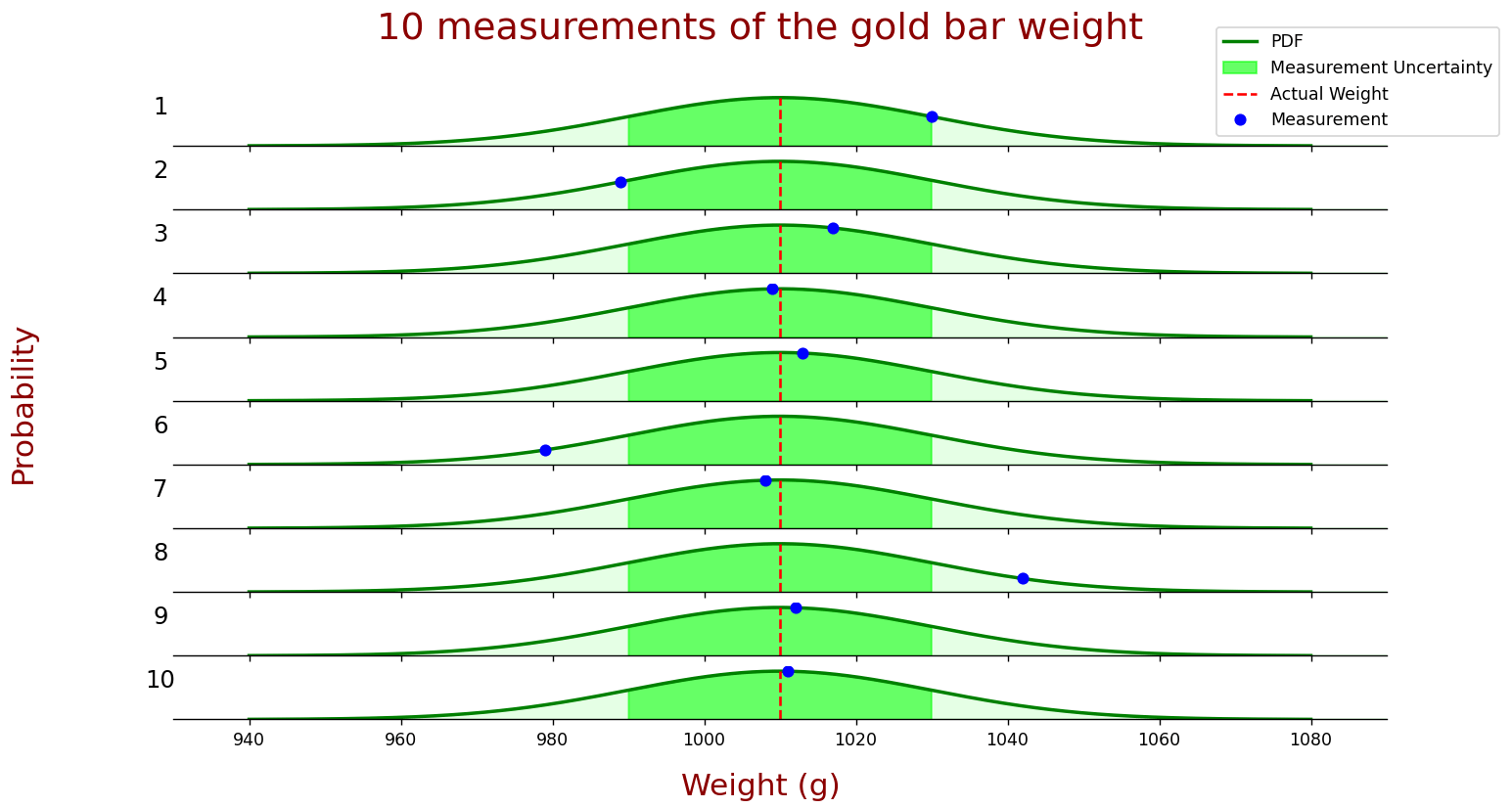### State prediction

In our first example, the measurement of the gold bar weight, the dynamic model is constant:

$\hat{x}_{n+1,n}= \hat{x}_{n,n}$

In the second example, the one-dimensional radar case, we extrapolated the current state (target position and velocity) to the next state using motion equations:

$\hat{x}_{n+1,n}= \hat{x}_{n,n}+ \Delta t\hat{\dot{x}}_{n,n}$ $\hat{\dot{x}}_{n+1,n}= \hat{\dot{x}}_{n,n}$

i.e., the predicted position equals the current estimated position plus the currently estimated velocity multiplied by the time. The predicted velocity equals the current velocity estimate (assuming a constant velocity model).

The dynamic model equation depends on the system.

Since Kalman Filter treats the estimate as a random variable, we must also extrapolate the estimation variance ( $$p_{n,n}$$ ) to the next state.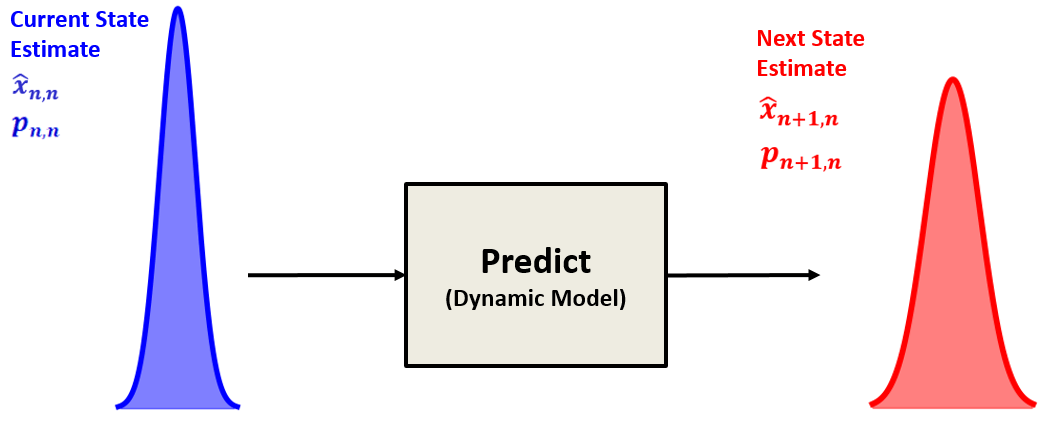In our first example (gold bar weight measurement), the dynamic model of the system is constant. Thus, the estimate uncertainty extrapolation would be:

$\hat{p}_{n+1,n}= \hat{p}_{n,n}$

Where:

$$p$$ is the estimate variance of the gold bar weight.

In the second example, the estimate uncertainty extrapolation would be:

$p_{n+1,n}^{x}= p_{n,n}^{x} + \Delta t^{2} \cdot p_{n,n}^{v}$ $p_{n+1,n}^{v}= p_{n,n}^{v}$
Where:
 $$p^{x}$$ is the position estimate variance $$p^{v}$$ is the velocity estimate variance

i.e., the predicted position estimate variance equals the current position estimate variance plus the current velocity estimate variance multiplied by the time squared. The predicted velocity estimate variance equals the current velocity estimate variance (assuming a constant velocity model).

Note that for any normally distributed random variable $$x$$ with variance $$\sigma^{2}$$, $$kx$$ is distributed normally with variance $$k^{2}\sigma^{2}$$, therefore the time term in the uncertainty extrapolation equation is squared. You can find a detailed explanation in the expectation of variance derivation appendix.

The estimate uncertainty extrapolation equation is called the Covariance Extrapolation Equation, which is the third Kalman Filter equation. Why covariance? We will see this in the multivariate Kalman Filter chapters.

### State update

To estimate the current state of the system, we combine two random variables:

• The prior state estimate (the current state estimate predicted at the previous state)
• The measurement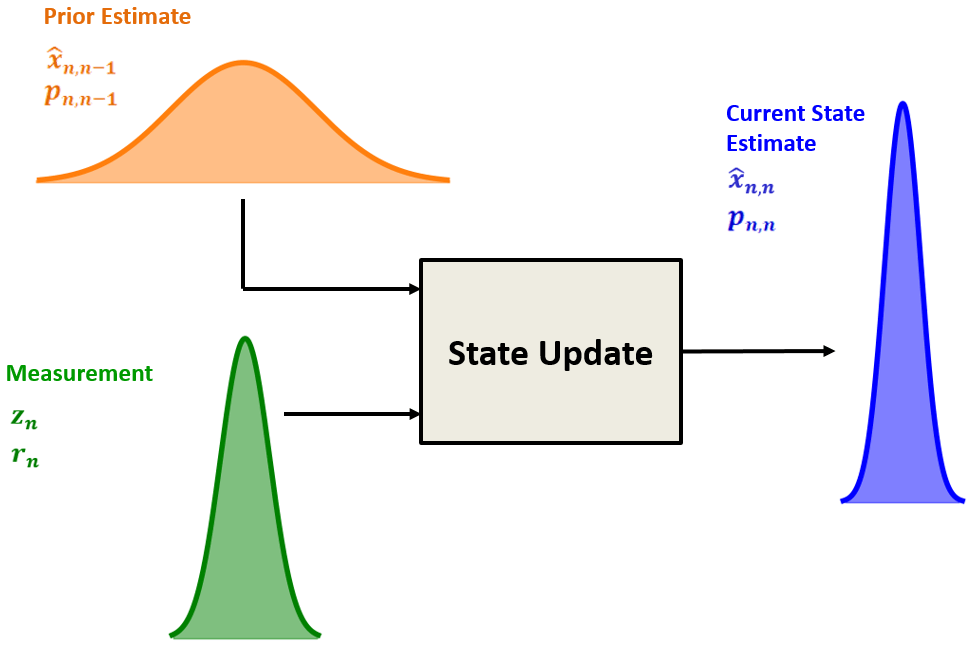The Kalman Filter is an optimal filter. It combines the prior state estimate with the measurement in a way that minimizes the uncertainty of the current state estimate.

The current state estimate is a weighted mean of the measurement and the prior state estimate:

$\hat{x}_{n,n} = w_{1}z_{n} + w_{2}\hat{x}_{n,n-1}$ $w_{1} + w_{2} = 1$

Where $$w_{1}$$ and $$w_{2}$$ are the weights of the measurement and the prior state estimate.

We can write $$\hat{x}_{n,n}$$ as follows:

$\hat{x}_{n,n} = w_{1}z_{n} + (1 - w_{1})\hat{x}_{n,n-1}$

The relation between variances is given by:

$p_{n,n} = w_{1}^{2}r_{n} + (1 - w_{1})^{2}p_{n,n-1}$

Where:

$$p_{n,n}$$ is the variance of the optimum combined estimate $$\hat{x}_{n,n}$$

$$p_{n,n-1}$$ is the variance of the prior estimate $$\hat{x}_{n,n-1}$$

$$r_{n}$$ is the variance of the measurement $$z_{n}$$

Remember that for any normally distributed random variable $$x$$ with variance $$\sigma^{2}$$, $$kx$$ is distributed normally with variance $$k^{2}\sigma^{2}$$. You can find a detailed explanation in the expectation of variance derivation appendix.

Since we are looking for an optimum estimate, we want to minimize $$p_{n,n}$$.

To find $$w_{1}$$ that minimizes $$p_{n,n}$$, we differentiate $$p_{n,n}$$ with respect to $$w_{1}$$ and set the result to zero.

$\frac{dp_{n,n}}{dw_{1}} = 2w_{1}r_{n} - 2(1 - w_{1})p_{n,n-1} = 0$

Hence

$w_{1}r_{n} = p_{n,n-1} - w_{1}p_{n,n-1}$

$w_{1}p_{n,n-1} + w_{1}r_{n} = p_{n,n-1}$

$w_{1} = \frac{p_{n,n-1}}{p_{n,n-1} + r_{n}}$

Let us substitute the result into the current state estimation $$\hat{x}_{n,n}$$:

$\hat{x}_{n,n} = w_{1}z_{n} + (1 - w_{1})\hat{x}_{n,n-1} = w_{1}z_{n} + \hat{x}_{n,n-1} - w_{1}\hat{x}_{n,n-1} = \hat{x}_{n,n-1} + w_{1}\left( z_{n} - \hat{x}_{n,n-1} \right)$

$\hat{x}_{n,n} = \hat{x}_{n,n-1} + \frac{p_{n,n-1}}{p_{n,n-1} + r_{n}}\left( z_{n} - \hat{x}_{n,n-1} \right)$

We have derived an equation that looks similar to the $$\alpha -\beta -(\gamma)$$ filter state update equation. The weight of the innovation is called the Kalman Gain (denoted by $$K_{n}$$).

The Kalman Gain Equation is the fourth Kalman Filter equation. In one dimension, the Kalman Gain Equation is the following:

$K_{n}= \frac{Variance \quad in \quad Estimate}{Variance \quad in \quad Estimate \quad + \quad Variance \quad in \quad Measurement}= \frac{p_{n,n-1}}{p_{n,n-1}+r_{n}}$
Where:
 $$p_{n,n-1}$$ is the extrapolated estimate variance $$r_{n}$$ is the measurement variance

The Kalman Gain is a number between zero and one:

$0 \leq K_{n} \leq 1$

Finally, we need to find the variance of the current state estimate. We've seen that the relation between variances is given by:

$p_{n,n} = w^{2}_{1}r_{n} + \left( 1 - w_{1} \right)^{2}p_{n,n-1}$

The weight $$w_{1}$$ is a Kalman Gain:

$p_{n,n} = K^{2}_{n}r_{n} + \left( 1 - K_{n} \right)^{2}p_{n,n-1}$

Let us find the $$\left( 1 - K_{n} \right)$$ term:

$\left( 1 - K_{n} \right) = \left( 1 - \frac{p_{n,n-1}}{p_{n,n-1} + r_{n}} \right) = \left( \frac{p_{n,n-1} + r_{n} - p_{n,n-1}}{p_{n,n-1} + r_{n}} \right) = \left( \frac{r_{n}}{p_{n,n-1} + r_{n}} \right)$

Notes
$$p_{n,n} = \left( \frac{p_{n,n-1}}{p_{n,n-1} + r_{n}} \right)^{2}r_{n} + \left( \frac{r_{n}}{p_{n,n-1} + r_{n}} \right)^{2}p_{n,n-1}$$ Substitute $$K_{n}$$ and $$\left( 1 - K_{n} \right)$$
$$p_{n,n} = \frac{p_{n,n-1}^{2}r_{n}}{\left(p_{n,n-1} + r_{n}\right)^{2}} + \frac{r_{n}^{2}p_{n,n-1}}{\left(p_{n,n-1} + r_{n}\right)^{2}}$$ Expand
$$p_{n,n} = \frac{p_{n,n-1}r_{n}}{p_{n,n-1} + r_{n}} \left( \frac{p_{n,n-1}}{p_{n,n-1} + r_{n}} + \frac{r_{n}}{p_{n,n-1} + r_{n}} \right)$$
$$p_{n,n} = \left( 1 - K_{n} \right)p_{n,n-1}\left( K_{n} + \left( 1 - K_{n} \right) \right)$$ Substitute $$K_{n}$$ and $$\left( 1 - K_{n} \right)$$
$$p_{n,n} = \left( 1 - K_{n} \right)p_{n,n-1}$$
$p_{n,n} = \left( 1 - K_{n} \right)p_{n,n-1}$

This equation updates the estimate variance of the current state. It is called the Covariance Update Equation.

It is clear from the equation that the estimate uncertainty is constantly decreasing with each filter iteration, since $$\left( 1-K_{n} \right) \leq 1$$. When the measurement uncertainty is high, the Kalman gain is low. Therefore, the convergence of the estimate uncertainty would be slow. However, the Kalman gain is high when the measurement uncertainty is low. Therefore, the estimate uncertainty would quickly converge toward zero.

So, it is up to us to decide how many measurements to make. If we are measuring a building height, and we are interested in a precision of 3 centimeters ( $$\sigma$$ ), we should make measurements until the state estimate variance ( $$\sigma ^{2}$$ ) is less than $$9~centimeters^{2}$$.

### Putting all together

This section combines all of these pieces into a single algorithm.

The filter inputs are:

• Initialization
• The initialization is performed only once, and it provides two parameters:

• Initial System State ( $$\hat{x}_{0,0}$$ )
• Initial State Variance ( $$p_{0,0}$$ )

The initialization parameters can be provided by another system, another process (for instance, a search process in radar), or an educated guess based on experience or theoretical knowledge. Even if the initialization parameters are not precise, the Kalman filter can converge close to the true value.

• Measurement
• The measurement is performed for every filter cycle, and it provides two parameters:

• Measured System State ( $$z_{n}$$ )
• Measurement Variance ( $$r_{n}$$ )

The filter outputs are:

• System State Estimate ( $$\hat{x}_{n,n}$$ )
• Estimate Variance ( $$p_{n,n}$$ )
The following table summarizes the five Kalman Filter equations.

Equation Equation Name Alternative names used in the literature
State Update $$\hat{x}_{n,n}=~ \hat{x}_{n,n-1}+ K_{n} \left( z_{n}- \hat{x}_{n,n-1} \right)$$ State Update Filtering Equation
$$p_{n,n}=~ \left( 1-K_{n} \right) p_{n,n-1}$$ Covariance Update Corrector Equation
$$K_{n}= \frac{p_{n,n-1}}{p_{n,n-1}+r_{n}}$$ Kalman Gain Weight Equation
State Predict $$\hat{x}_{n+1,n}= \hat{x}_{n,n}$$
(For constant dynamics)

$$\hat{x}_{n+1,n}= \hat{x}_{n,n}+ \Delta t\hat{\dot{x}}_{n,n}$$
$$\hat{\dot{x}}_{n+1,n}= \hat{\dot{x}}_{n,n}$$
(For constant velocity dynamics)
State Extrapolation Predictor Equation
Transition Equation
Prediction Equation
Dynamic Model
State Space Model
$$p_{n+1,n}= p_{n,n}$$
(For constant dynamics)

$$p_{n+1,n}^{x}= p_{n,n}^{x} + \Delta t^{2} \cdot p_{n,n}^{v}$$
$$p_{n+1,n}^{v}= p_{n,n}^{v}$$
(For constant velocity dynamics)
Covariance Extrapolation Predictor Covariance Equation
Note 1: The equations above don't include the process noise. In the following chapter, we add process noise.
Note 2: The State Extrapolation Equation and the Covariance Extrapolation Equation depend on the system dynamics.
Note 3: The table above demonstrates a special form of the Kalman Filter equations tailored for a specific case. The general form of the equation is presented later in matrix notation. Right now, our goal is to understand the concept of the Kalman Filter.

The following figure provides a detailed description of the Kalman Filter's block diagram.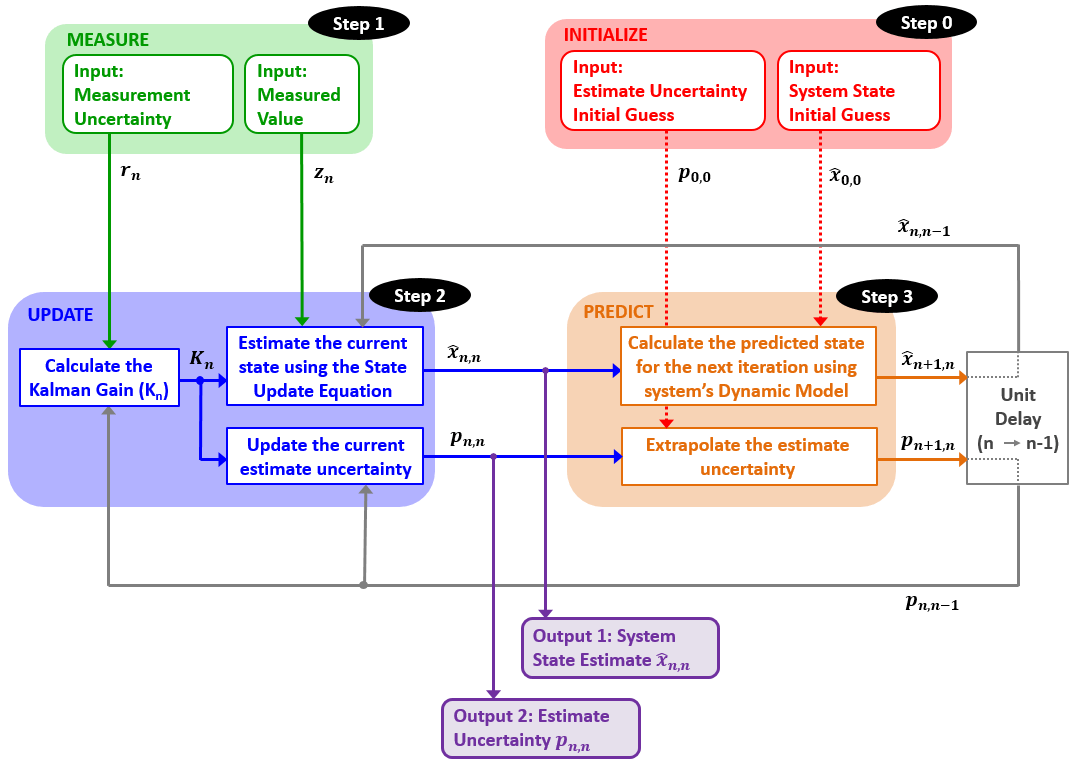• Step 0: Initialization
• As mentioned above, the initialization is performed only once, and it provides two parameters:

• Initial System State ( $$\hat{x}_{0,0}$$ )
• Initial State Variance ( $$p_{0,0}$$ )

The initialization is followed by prediction.

• Step 1: Measurement
• The measurement process provides two parameters:

• Measured System State ( $$z_{n}$$ )
• Measurement Variance ( $$r_{n}$$ )
• Step 2: State Update
• The state update process is responsible for the state estimation of the current state of the system.

The state update process inputs are:

• Measured Value ( $$z_{n}$$ )
• A Measurement Variance ( $$r_{n}$$ )
• A prior Predicted System State Estimate ( $$\hat{x}_{n,n-1}$$ )
• A prior Predicted System State Estimate Variance ( $$p_{n,n-1}$$ )

Based on the inputs, the state update process calculates the Kalman Gain and provides two outputs:

• Current System State Estimate ( $$\hat{x}_{n,n}$$ )
• Current State Estimate Variance ( $$p_{n,n}$$ )

These parameters are the Kalman Filter outputs.

• Step 3: Prediction
• The prediction process extrapolates the current system state estimate and its variance to the next system state based on the dynamic model of the system.

At the first filter iteration, the initialization is treated as the Prior State Estimate and Variance.

The prediction outputs are used as the Prior (predicted) State Estimate and Variance on the following filter iterations.

### Kalman Gain intuition

Let's rewrite the state update equation:

$\hat{x}_{n,n} = \hat{x}_{n,n-1} + K_{n}\left( z_{n} - \hat{x}_{n,n-1} \right) = \left( 1 - K_{n} \right)\hat{x}_{n,n-1} + K_{n}z_{n}$

As you can see, the Kalman Gain ( $$K_{n}$$ ) is the measurement weight, and the $$\left( 1 - K_{n} \right)$$ term is the weight of the current state estimate.

The Kalman Gain is close to zero when the measurement uncertainty is high and the estimate uncertainty is low. Hence we give a significant weight to the estimate and a small weight to the measurement.

On the other hand, when the measurement uncertainty is low, and the estimate uncertainty is high, the Kalman Gain is close to one. Hence we give a low weight to the estimate and a significant weight to the measurement.

If the measurement uncertainty equals the estimate uncertainty, then the Kalman gain equals 0.5.

The Kalman Gain Defines the measurement's weight and the prior estimate's weight when forming a new estimate. It tells us how much the measurement changes the estimate.

#### High Kalman Gain

A low measurement uncertainty relative to the estimate uncertainty would result in a high Kalman Gain (close to 1). Therefore the new estimate would be close to the measurement. The following figure illustrates the influence of a high Kalman Gain on the estimate in an aircraft tracking application.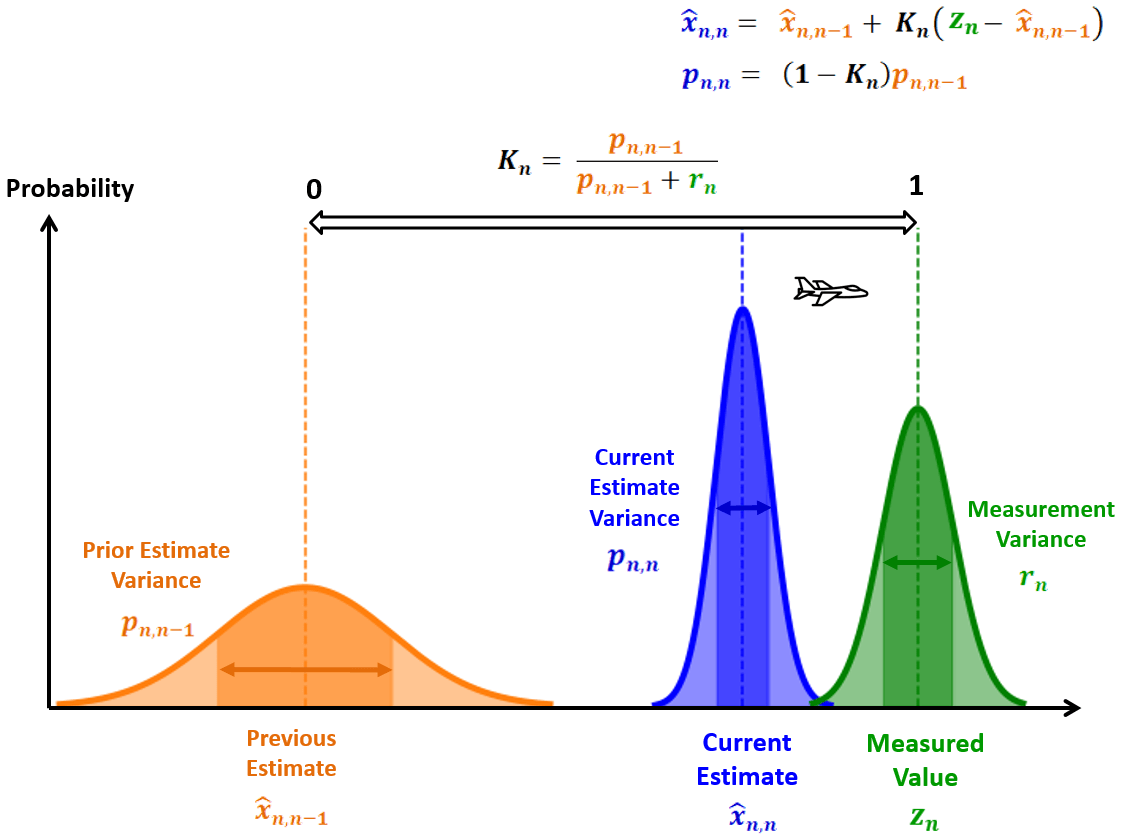#### Low Kalman Gain

A high measurement uncertainty relative to the estimate uncertainty would result in a low Kalman Gain (close to 0). Therefore the new estimate would be close to the prior estimate. The following figure illustrates the influence of a low Kalman Gain on the estimate in an aircraft tracking application.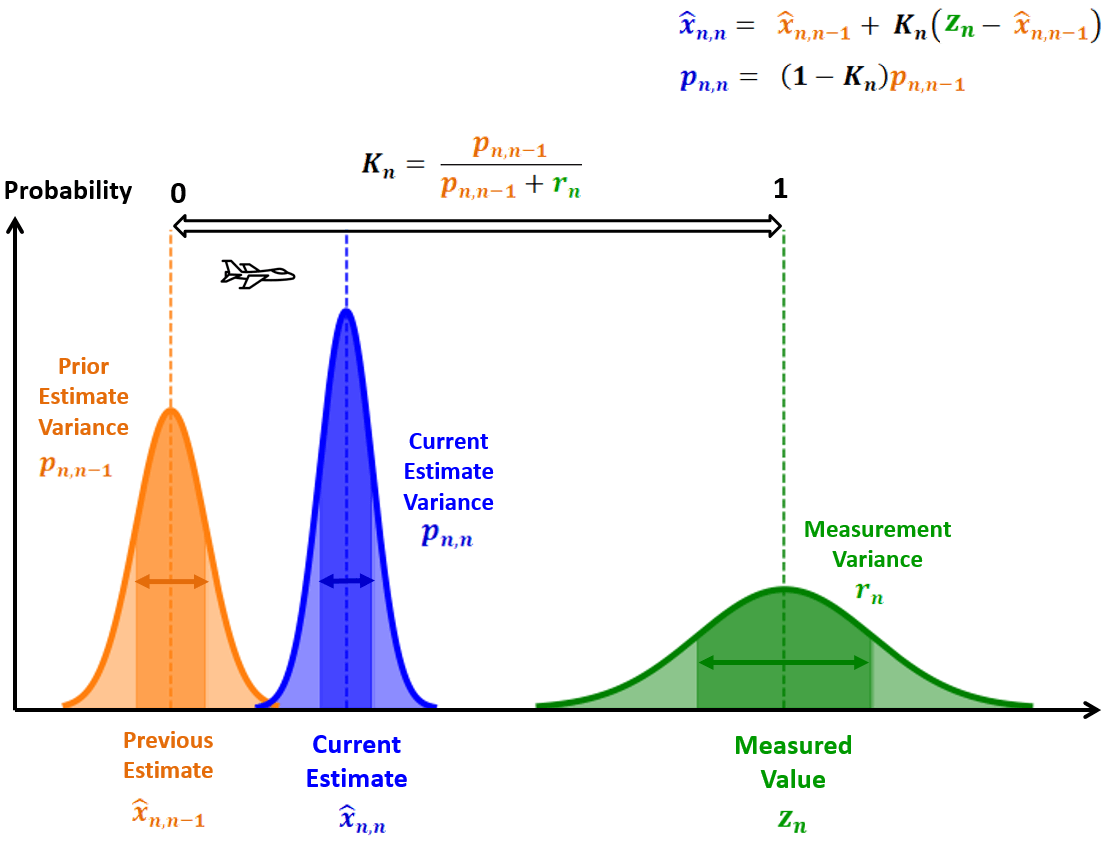Now we understand the Kalman Filter algorithm and are ready for the first numerical example.

## Example 5 – Estimating the height of a building

Assume that we would like to estimate the height of a building using an imprecise altimeter.

We know that building height doesn't change over time, at least during the short measurement process.### The numerical example

• The true building height is 50 meters.
• The altimeter measurement error (standard deviation) is 5 meters.
• The ten measurements are: 49.03m, 48.44m, 55.21m, 49.98m, 50.6m, 52.61m, 45.87m, 42.64m, 48.26m, 55.84m.

#### Iteration Zero

##### Initialization

One can estimate the height of the building simply by looking at it.

The estimated height of the building for the initialization purpose is:

$\hat{x}_{0,0}=60m$

Now we shall initialize the estimate variance. A human estimation error (standard deviation) is about 15 meters: $$\sigma =15$$ . Consequently the variance is 225: $$\sigma ^{2}=225$$.

$p_{0,0} = 225$

##### Prediction

Now, we shall predict the next state based on the initialization values.

Since our system's Dynamic Model is constant, i.e., the building doesn't change its height:

$\hat{x}_{1,0}=\hat{x}_{0,0}= 60m$

The extrapolated estimate variance also doesn't change:

$p_{1,0}= p_{0,0}=225$

#### First Iteration

##### Step 1 - Measure

The first measurement is: $$z_{1}=49.03m$$ .

Since the standard deviation ( $$\sigma$$ ) of the altimeter measurement error is 5, the variance ( $$\sigma ^{2}$$ ) would be 25, thus, the measurement variance is: $$r_{1}=25$$ .

##### Step 2 - Update

Kalman Gain calculation:

$K_{1}= \frac{p_{1,0}}{p_{1,0}+r_{1}}= \frac{225}{225+25}=0.9$

Estimating the current state:

$\hat{x}_{1,1}=~ \hat{x}_{1,0}+ K_{1} \left( z_{1}- \hat{x}_{1,0} \right) =60+0.9 \left( 49.03-60 \right) =50.13m$

Update the current estimate variance:

$p_{1,1}=~ \left( 1-K_{1} \right) p_{1,0}= \left( 1-0.9 \right) 225=22.5$

##### Step 3 - Predict

Since the dynamic model of our system is constant, i.e., the building doesn't change its height:

$\hat{x}_{2,1}=\hat{x}_{1,1}= 50.13m$

The extrapolated estimate variance also doesn't change:

$p_{2,1}= p_{1,1}=22.5$

#### Second Iteration

After a unit time delay, the predicted estimate from the previous iteration becomes the prior estimate in the current iteration:

$\hat{x}_{2,1}=50.13m$

The extrapolated estimate variance becomes the prior estimate variance:

$p_{2,1}= 22.5$

##### Step 1 - Measure

The second measurement is: $$z_{2} = 48.44m$$

The measurement variance is: $$r_{2}=25$$

##### Step 2 - Update

Kalman Gain calculation:

$K_{2}= \frac{p_{2,1}}{p_{2,1}+r_{2}}= \frac{22.5}{22.5+25}=0.47$

Estimating the current state:

$\hat{x}_{2,2}=~ \hat{x}_{2,1}+ K_{2} \left( z_{2}- x_{2,1} \right) =50.13+0.47 \left( 48.44-50.13 \right) =49.33m$

Update the current estimate variance:

$p_{2,2}=~ \left( 1-K_{2} \right) p_{2,1}= \left( 1-0.47 \right) 22.5=11.84$

##### Step 3 - Predict

Since the dynamic model of our system is constant, i.e., the building doesn't change its height:

$\hat{x}_{3,2}=\hat{x}_{2,2}= 49.33m$

The extrapolated estimate variance also doesn't change:

$p_{3,2}= p_{2,2}=11.84$

#### Iterations 3-10

The calculations for the subsequent iterations are summarized in the following table:

$$n$$ $$z_{n}$$ Current state estimates ( $$K_{n}$$ , $$\hat{x}_{n,n}$$ , $$p_{n,n}$$ ) Prediction ( $$\hat{x}_{n+1,n}$$ , $$p_{n+1,n}$$ )
3 $$55.21m$$ $K_{3}= \frac{11.84}{11.84+25}=0.32$ $\hat{x}_{3,3}=~ 49.33+0.32 \left( 55.21 -49.33 \right) =51.22m$ $p_{3,3}= \left( 1-0.32 \right) 11.84=8.04$ $\hat{x}_{4,3}= \hat{x}_{3,3}=51.22m$ $p_{4,3}= p_{3,3}=8.04$
4 $$49.98m$$ $K_{4}= \frac{8.04}{8.04+25}=0.24$ $\hat{x}_{4,4}= 51.22+0.24 \left( 49.98 -51.22 \right) =50.92m$ $p_{4,4}= \left( 1-0.24 \right) 8.04=6.08$ $\hat{x}_{5,4}= \hat{x}_{4,4}=50.92m$ $p_{5,4}= p_{4,4}=6.08$
5 $$50.6m$$ $K_{5}= \frac{6.08}{6.08+25}=0.2$ $\hat{x}_{5,5}= 50.92+0.2 \left( 50.6 -50.92 \right) =50.855m$ $p_{5,5}= \left( 1-0.2 \right) 6.08=4.89$ $\hat{x}_{6,5}= \hat{x}_{5,5}=50.855m$ $p_{6,5}= p_{5,5}=4.89$
6 $$52.61m$$ $K_{6}= \frac{4.89}{4.89+25}=0.16$ $\hat{x}_{6,6}=~ 50.855+0.16 \left( 52.61 -50.855 \right) =51.14m$ $p_{6,6}= \left( 1-0.16 \right) 4.89=4.09$ $\hat{x}_{7,6}= \hat{x}_{6,6}=51.14m$ $p_{7,6}= p_{6,6}=4.09$
7 $$45.87m$$ $K_{7}= \frac{4.09}{4.09+25}=0.14$ $\hat{x}_{7,7}= 51.14+0.14 \left( 45.87 -51.14 \right) =50.4m$ $p_{7,7}= \left( 1-0.14 \right) 4.09=3.52$ $\hat{x}_{8,7}= \hat{x}_{7,7}=50.4m$ $p_{8,7}= p_{7,7}=3.52$
8 $$42.64m$$ $K_{8}= \frac{3.52}{3.52+25}=0.12$ $\hat{x}_{8,8}= 50.4+0.12 \left( 42.64 -50.4 \right) =49.44m$ $p_{8,8}= \left( 1-0.12 \right) 3.52=3.08$ $\hat{x}_{9,8}= \hat{x}_{8,8}=49.44m$ $p_{9,8}= p_{8,8}=3.08$
9 $$48.26m$$ $K_{9}= \frac{3.08}{3.08+25}=0.11$ $\hat{x}_{9,9}= 49.44 + 0.11 \left( 48.26 - 49.44 \right) = 49.31m$ $p_{9,9}= \left( 1-0.11 \right) 3.08=2.74$ $\hat{x}_{10,9}= \hat{x}_{9,9} = 49.31m$ $p_{10,9}= p_{9,9}=2.74$
10 $$55.84m$$ $K_{10}= \frac{2.74}{2.74+25}=0.1$ $\hat{x}_{10,10}= 49.31 + 0.1 \left( 55.84 - 49.31 \right) = 49.96m$ $p_{10,10}= \left( 1-0.1 \right) 2.74=2.47$ $\hat{x}_{11,10}= \hat{x}_{10,10} = 49.96m$ $p_{11,10}= p_{10,10} = 2.47$

### Results analysis

First of all, we want to ensure Kalman Filter convergence. The Kalman Gain should gradually decrease until it reaches a steady state. When Kalman Gain is low, the weight of the noisy measurements is also low. The following plot describes the Kalman Gain for the first one hundred iterations of the Kalman Filter.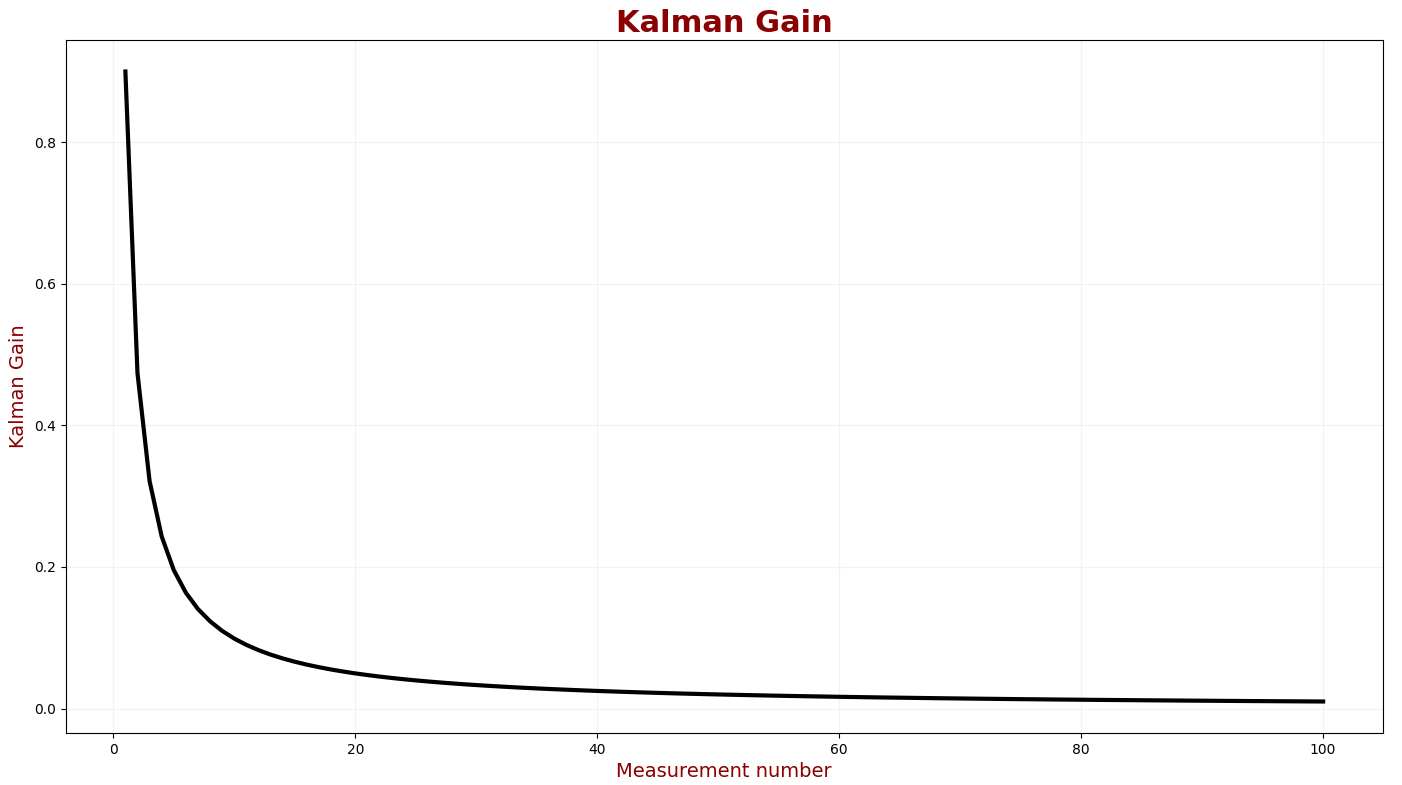We can see a significant reduction in the Kalman Gain during the first ten iterations. The Kalman Gain enters a steady state after approximately fifty iterations.

We also want to examine accuracy. Accuracy indicates how close the measurement is to the true value. The following chart compares the true value, measured values, and estimates for the first 50 iterations.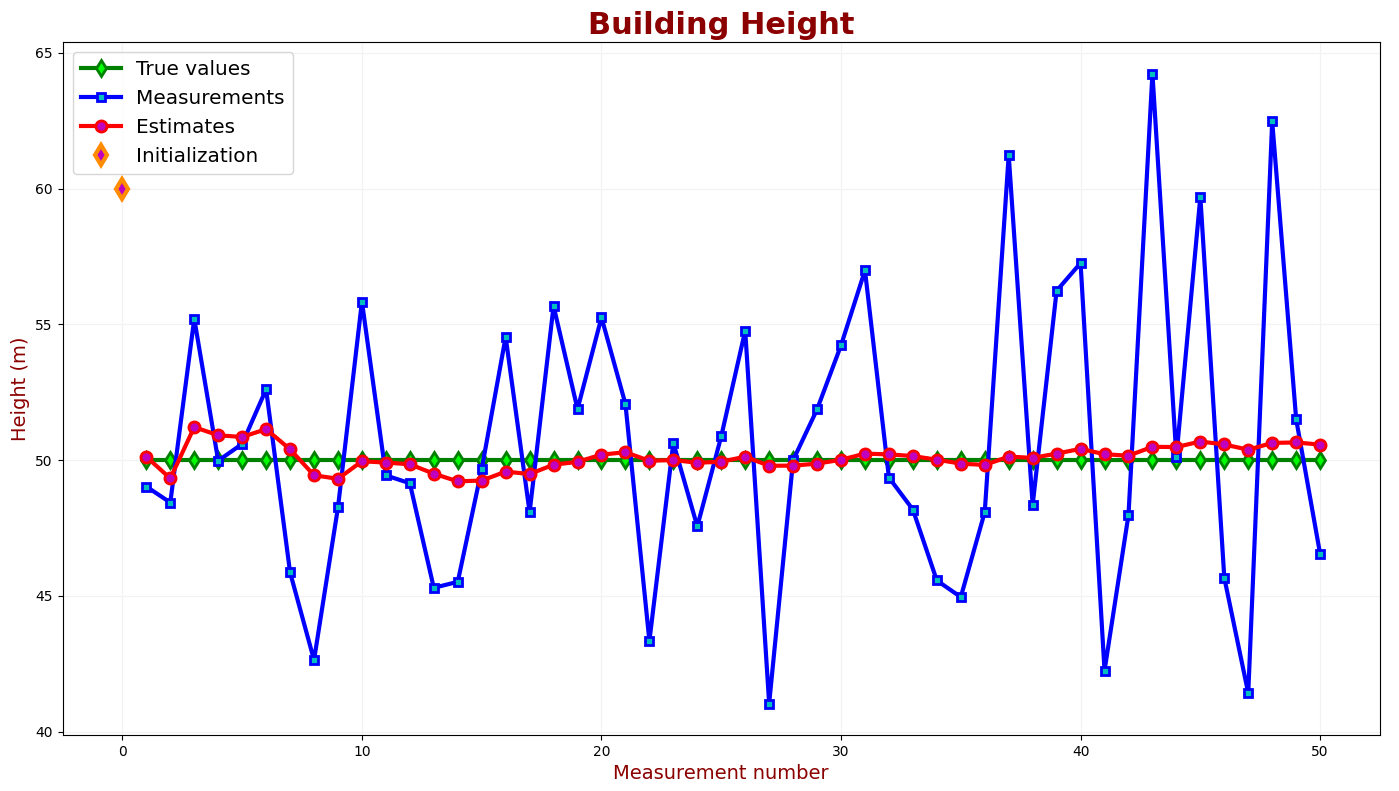An estimation error is a difference between the true values (the green line) and the KF estimates (the red line). We can see that the estimation errors of our KF decrease in the filter convergence region.

One can define accuracy criteria based on the specific application requirements. The typical accuracy criteria are:

• Maximum error
• Mean error
• RMSE(Root Mean Square Error)

Another important parameter is estimation uncertainty. We want the KF estimates to be precise; therefore, we are interested in low estimation uncertainty.

Assume that for a building height measurement application, there is a requirement for 95% confidence. The following chart shows the KF estimates and the true values with 95% confidence intervals.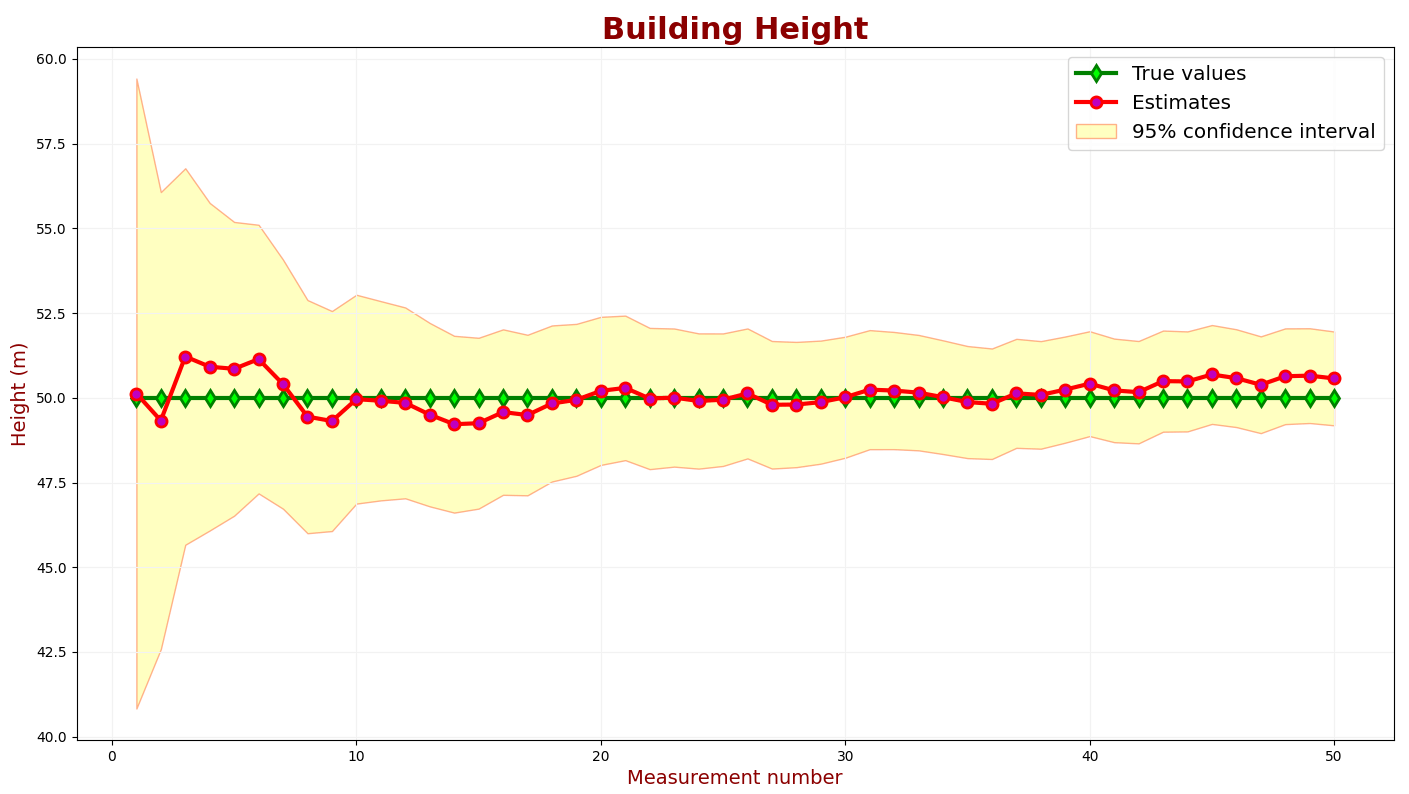The confidence intervals are calculated based on the uncertainties of the estimates. You can find the guidelines for a confidence interval calculation here.

In the above chart, the confidence intervals are added to the estimates (the red line). 95% of the green samples should be within the 95% confidence region.

We can see that the uncertainty is too high. Let us decrease the measurement uncertainty.

The following chart describes the KF output for a low measurement uncertainty parameter.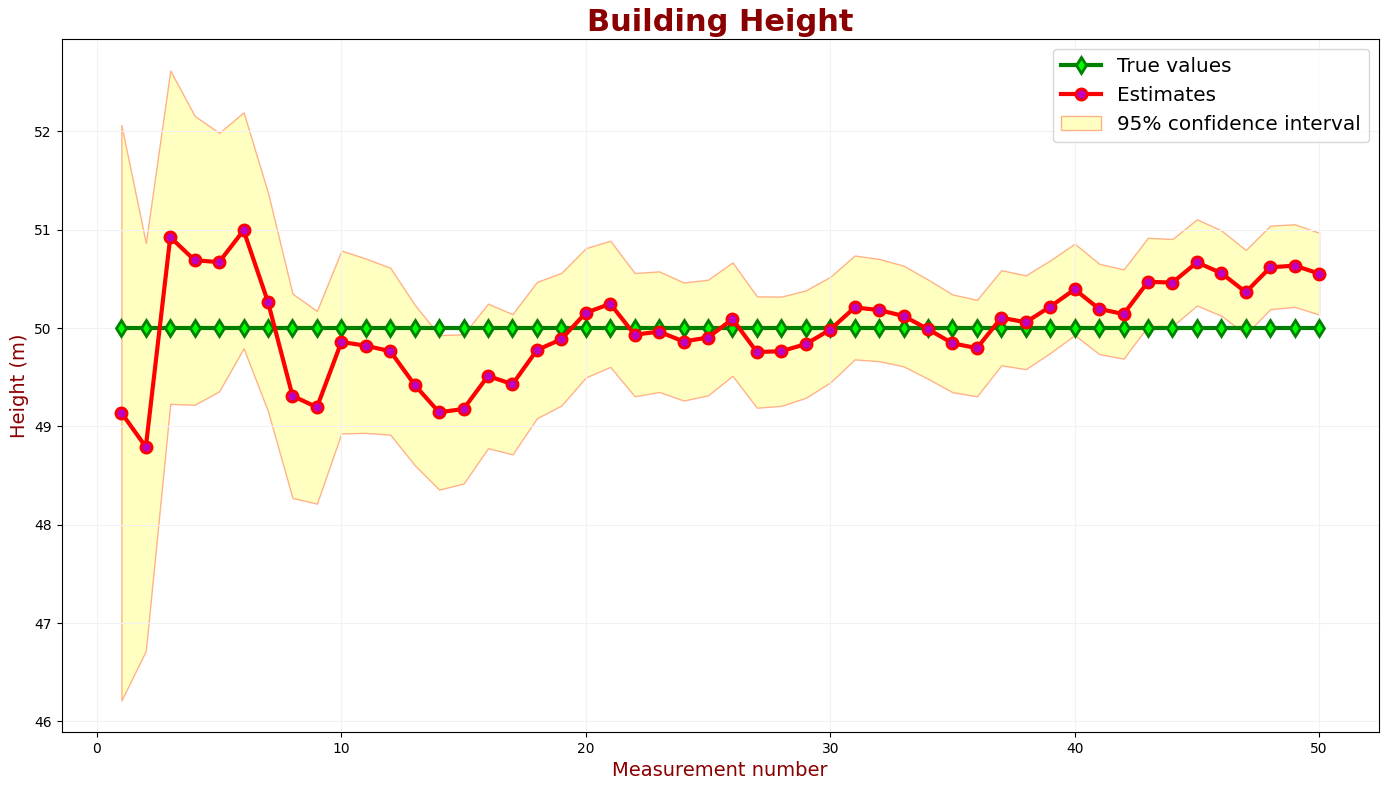Although we've decreased the uncertainty of the estimates, many green samples are outside the 95% confidence region. The Kalman Filter is overconfident and too optimistic about its accuracy.

Let us find the measurement uncertainty that yields the desired estimate uncertainty.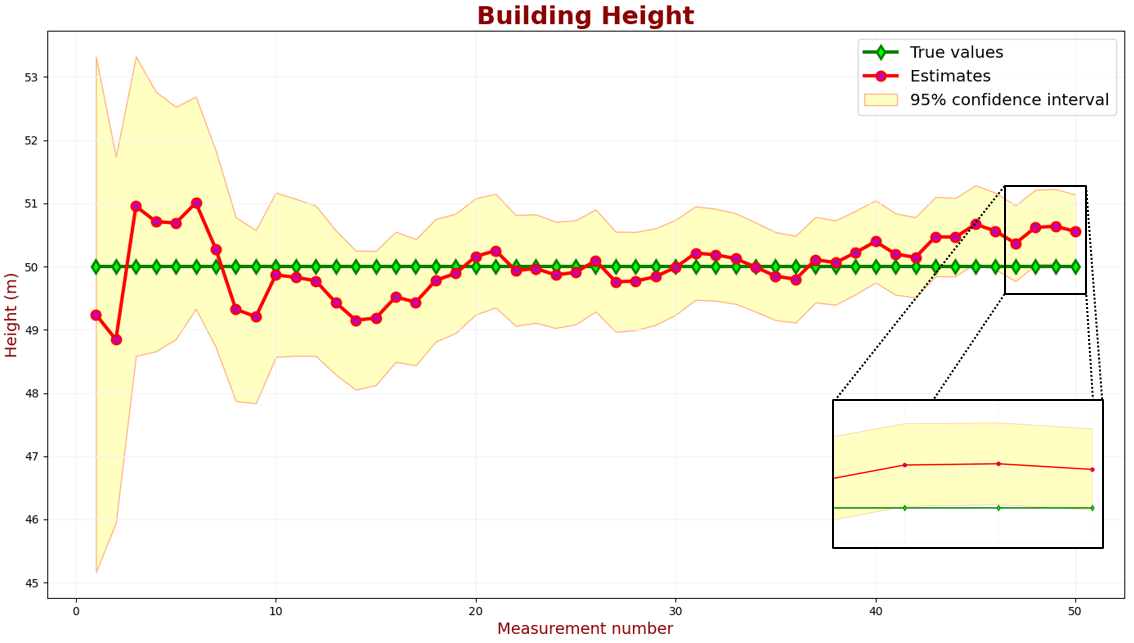The above chart shows that 2 out of 50 samples slightly exceed the 95% confidence region. This performance satisfies our requirements.

### Example summary

We measured the building height using the one-dimensional Kalman Filter in this example. Unlike the $$\alpha -\beta -(\gamma)$$ filter, the Kalman Gain is dynamic and depends on the precision of the measurement device.

The initial value used by the Kalman Filter is not precise. Therefore, the measurement weight in the State Update Equation is high, and the estimate uncertainty is high.

With each iteration, the measurement weight is lower; therefore, the estimate uncertainty is lower.

The Kalman Filter output includes the estimate and the estimate uncertainty.